International
Tables for
Crystallography
Volume F
Crystallography of biological macromolecules
Edited by M. G. Rossmann and E. Arnold

International Tables for Crystallography (2006). Vol. F, ch. 11.3, pp. 223-224   | 1 | 2 |

## Section 11.3.5. Post refinement

W. Kabscha*

aMax-Planck-Institut für medizinische Forschung, Abteilung Biophysik, Jahnstrasse 29, 69120 Heidelberg, Germany
Correspondence e-mail: kabsch@mpimf-heidelberg.mpg.de

### 11.3.5. Post refinement

| top | pdf |

The number of fully recorded reflections on each single image rapidly declines for small oscillation ranges and the complete intensities of the partially recorded reflections have to be estimated. This presented a serious obstacle in early structural work on virus crystals, as the crystal had to be replaced after each exposure on account of radiation damage. A solution of this problem, the post refinement' technique, was found by Schutt, Winkler and Harrison, and variants of this powerful method have been incorporated into most data-reduction programs [for a detailed discussion, see Harrison et al. (1985); Rossmann (1985)]. The method derives complete intensities of reflections only partially recorded on an image from accurate estimates for the fractions of observed intensity, the partiality'. The partiality of each reflection can always be calculated as a function of orientation, unit-cell metric, mosaic spread of the crystal and model intensity distributions. Obviously, the accuracy of the estimated full reflection intensity then strongly depends on a precise knowledge of the parameters describing the diffraction experiment. Usually, for many of the partial reflections, symmetry-related fully recorded ones can be found, and the list of such pairs of intensity observations can be used to refine the required parameters by a least-squares procedure. Clearly, this refinement is carried out after all images have been processed, which explains why the procedure is called `post refinement'.

Adjustments of the diffraction parameters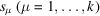are determined by minimization of the function E, which is defined as the weighted sum of squared residuals between calculated and observed partial intensites.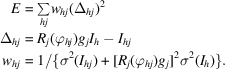Here,is the intensity recorded on image j of a partial reflection with indices summarized as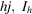is the mean of the observed intensities of all fully recorded reflections symmetry-equivalent to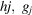is the inverse scaling factor of image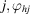is the calculated spindle angle of reflection hj at diffraction and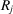is the computed fraction of total intensity recorded on image j.

Expansion of the residuals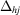to first order in the parameter changes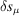and minimization of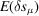leads to the k normal equations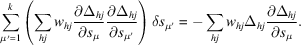Often, the normal matrix is ill-conditioned, since changes in some unit-cell parameters or small rotations of the crystal about the incident X-ray beam do not significantly affect the calculated partiality. To take care of these difficulties, the system of equations is rescaled to yield unit diagonal elements for the normal matrix and the correction vectoris filtered by projection into a subspace defined by the eigenvectors of the normal matrix with sufficiently large eigenvalues (Diamond, 1966).

The parameters are corrected by the filteredand a new cycle of refinement is started until a minimum of E is reached. The weights, residuals and their gradients are calculated using the current values for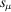andat the beginning of each cycle. The derivatives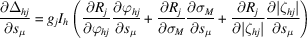appearing in the normal equations can be worked out from the definitions given in Sections 11.3.2.2and 11.3.2.4(to simplify the following equations, the subscript hj is omitted). The fractionof the total intensity can be expressed in terms of the error function (see Section 11.3.2.4) as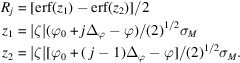Using the relation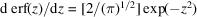, the derivatives ofare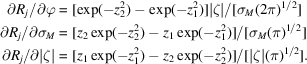It remains to work out the derivatives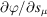,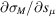and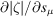(not shown here). As discussed in detail by Greenhough & Helliwell (1982), spectral dispersion and asymmetric beam cross fire lead to some variation of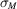, which makes it necessary to include additional parameters in the list. The effect of these parameters on the partiality is dealt with easily by the derivatives.

The refinement scheme described above requires initial scaling factors. With the now improved estimates for the partialities, a new set of scaling factors can be obtained by the method outlined in Section 11.3.4. This alternating procedure of scaling and post refinement usually converges within three cycles.

The use of error functions for modelling partiality, as implicated by a Gaussian model for describing spot shape, was chosen here for reasons of conceptual simplicity and coherence. This choice is unlikely to alter significantly the results of post refinement that are based on other functions of similar form [see the discussion by Rossmann (1985)].

### References

Diamond, R. (1966). A mathematical model-building procedure for proteins. Acta Cryst. 21, 253–266.Google Scholar
Greenhough, T. J. & Helliwell, J. R. (1982). Oscillation camera data processing: reflecting range and prediction of partiality. I. Conventional X-ray sources. J. Appl. Cryst. 15, 338–351.Google Scholar
Harrison, S. C., Winkler, F. K., Schutt, C. E. & Durbin, R. M. (1985). Oscillation method with large unit cells. Methods Enzymol. 114A, 211–237.Google Scholar
Rossmann, M. G. (1985). Determining the intensity of Bragg reflections from oscillation photographs. Methods Enzymol. 114A, 237–280.Google Scholar